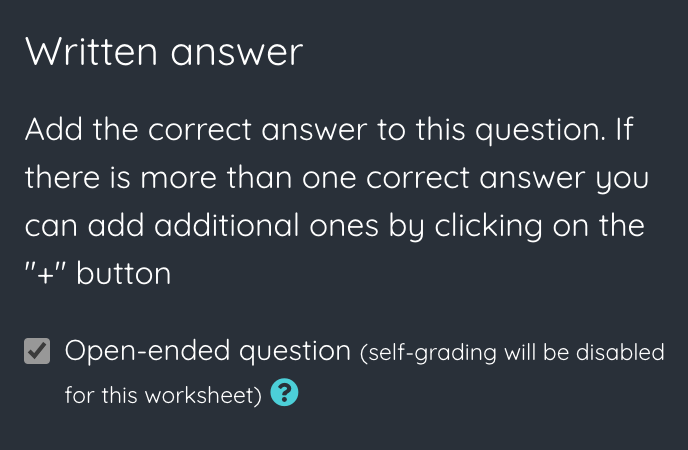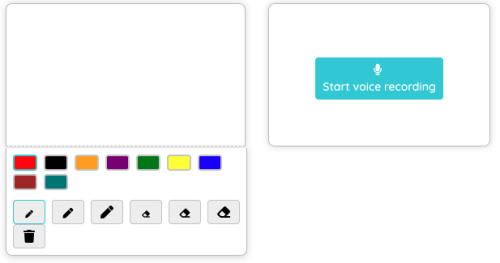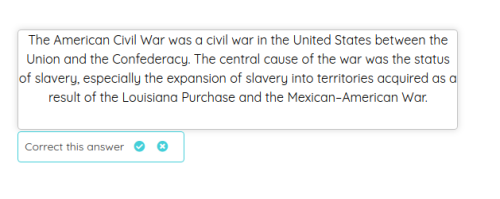# How to add open ended questions

In some worksheets we want to include questions that do not have a pre-set correct answer. In these questions the student can write a broader answer that must be corrected later by the teacher to indicate whether or not the answer is correct. For example, we can add a video to the worksheet and a question where we ask the student to summarize the content of the video.

We call these questions open-ended questions and we can add them very easily from the editor.To do this, we just have to add to the worksheet a field of type Written answer or Formula answer and tick the Open-ended question checkbox. This will hide the correct answer boxes and the question will become open-ended, so it will not be auto-graded and we will have to correct it manually when students send their answers.In addition, there are other types of fields that are also considered open-ended questions: the Draw field, the Voice answer, Image answer and Video answer fields. In those cases, it is not possible to correct automatically the student's answer, so they must be corrected manually.

When students finish a worksheet containing open questions they will not be shown the final grade, since it is not possible to autocorrect open questions. In this case, the teacher must correct the answers before being able to calculate the final grade.« Previous